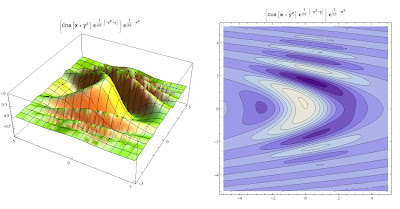## Sunday, August 12, 2012

### Function minimization: Simulated Annealing led by variance criteria vs Nelder Mead

Most of the datamining problems can be reduced as a minimization/maximization problem.
Whenever you are looking for the best trade off between costs and benefits you are solving a minimization problem.
Often the number of the variables that affects the cost function is high and the domain of these variables is in a dense set and, last but not least: in the real world problem often, you have no clue about the analytical form of the function.
Formally such problems belong to the multidimensional unconstrained optimization family.
Examples
Let's consider  easy scenarios where the function cost is conditionated just by two parameters.Schaffer f6 function: in the contour plot  dark purple to depict local minimums.Another function where minimum ischaracterized by two valleysThis function presents several local minimums well depicted in the contour plotThis function is characterized by a several local minimums having high range of values

As you can see the above problems have in common a massive presence of local minimum :).
Let's see how to handle these problems through an approach that I define hybrid, that is, obtained mixing different methods and properties.
Simulated Annealing (SA) "variance based" approach
Disclaimer: I had no the time to check whether this method has been already published somewhere. The underlying idea is quite simple, so I would assume that someone has already spent time in proofing convergence and better variations (and actually I don't think is a rocket science proof it).
SA, belongs to the family of numerical method based on "search strategy" and its convergence requirements are related to the stationary conditions inducted by the underlying markovian process.
In a former post I showed an its application in a discrete domain, where at each iteration we chose the next candidate solution by comparison with the current solution. The new solution was found "fishing randomly" a candidate in a discrete space.
In the above problems the solution space is continuos, so we need a smart strategy to extract the new candidate. Let's see my approach!
Variance trick
Let's consider a sampling in two different region of a function f :
• sampling_1 in a region having a smooth minimum and evaluate such points
• sampling_2 in a region having a spiky minimum and evaluate such points
Take now the variance of these evaluated sets.
How does the variance of f(sampling_1)  change respect the variance of f(sampling_2)?
As you can see the variance can be used as indicator of a minimum region of the cost function.
Instead of explore randomly the solution space, the approach I propose is led by the variance used as  above.
What happens if the smooth valley is a global minimum?
There are two intuitive factors to avoid that the algorithm jams in a local minimum:
1. The acceptance law admit also pejorative solutions.
2. The sampling procedure moderate by variance (if the variance is chosen high enough) allows explorations of better regions.

The algorithmThe meta code of the Simulated Annealing based on variance criteria
Many optimizations can be done to the algorithm.
For instance we could condition the annealing procedure only if the
argMin(evaluate(f,newSet)) > argMin(evaluate(f,curset))

Tests
Let's see how the approach works.
As usual, all my experiments are compared with other techniques. In this case I chose as comparative method the well known "Nelder Mead" method.
I tried to optimize the Nelder Mead method playing around its param setting: shrink ratio, contraction ratio and reflection ratio.
All experiments have been done using the same initial points.
For the Nelder Mead method I plotted just the unique solutions found.
The approach I propose has been led using always the same setting (3000 cycles, variance sampling = 0.75).
Experiment 1.On the left side it has been represented the solution found by Nelder Mead method. On the right side the solution found by the SA presented in this post: the blue point depicts the starting point and the red point depicts the solution found. The last chart shows the space solution explored.
Notice that method found a better solution respect Nelder Mead approach.

Experiment 2On the left side it has been represented the solution found by Nelder Mead method. On the right side the solution found by the SA presented in this post: the blue point depicts the starting point and the red point depicts the solution found.
SA found a better solution in really few steps.

Experiment 3On the left side it has been represented the solution found by Nelder Mead method. On the right side the solution found by the SA presented in this post: the blue point depicts the starting point and the red point depicts the solution found.
This experiment shows once again the good performance of this approach even if the problem is very challenging!

Experiment 4On the left side it has been represented the solution found by Nelder Mead method. On the right side the solution found by the SA presented in this post: the blue point depicts the starting point and the red point depicts the solution found.
The convergence, as shown above, has been reached very quickly.

Conclusion
The approach seems promising and works well in different contexts:  I'm quite sure that many improvements can be implemented.
1.